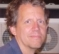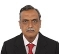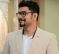## 16-bit number to LCD digitsStarted by 5 years ago6 replieslatest reply 5 years ago352 views

I'm trying to figure out the fastest way to convert a 16-bit number to 4 digits for an LCD display.

I did adopt the use of the fast 16-bit division on another thread here, but I think somehow I can get something faster.

If I was only interested in two digits from an 8-bit binary number, I could get away with this:

Assume R3 is the input number and R2:R1 are the output digits (R2=tens digit, R1=ones digit)

mov B,#10
mov A,R3
DIV AB
mov R1,B
mov B,#10
DIV AB
mov R2,B

But what happens if I wanted to try to display all digits from a 16-bit binary number?
For example, what if I wanted to show number 256 on the LCD? I can't with the code above because the above code would output 0 and 0 (because one input register will hold 01h and the other input register will hold 00h) for R1 and R2, and I definitely don't want to see 00 when I expect 256 printed.

Assume R4:R3 is input number. I know this is incomplete but I'm trying to figure it out.

mov ??,R4
??
mov B,#10
mov A,R3
DIV AB
mov R1,B
mov B,#10
DIV AB
mov R2,B

This person thinks he has a solution, but I don't quite understand it, especially when his comments partly don't make sense and also he is missing a label or has placed a label incorrectly.

https://okashtein.wordpress.com/2013/04/15/binary1...

[ - ]You could try counting the number of times you can subtract 10000, 1000, 100, and 10 from the original number.  You'd need a table containing the values 10000, 1000, 100, 10, and 1 (all 16 bit values), and a pointer to the start of the table.  Then starting with the first table value, count the number of times you can subtract 10000 until you get a value less than 10000.  This will be the 10000 digit.  Advance the table pointer by 2 and do the next digit with the remainder from the previous run.  Lather, rinse, repeat.  When you hit 1 in the table, the remainder will be the ones digit.

The nice thing is that you can use the digits in order from left to right, instead of getting them from right to left through division.

[ - ]Ok, so I did your trick and expanded things to 24-bits. I tried worst case scenario for my situation of the number 999,999 and my simulator indicated it takes 1,260 system clocks just to separate the digits into each individual memory location. That's alot of time spent on that. I gotta condense this somehow.

org 0h
mathtest:
mov SP,#70h
;R7:R6:R5 = input number: 999999
mov R7,#00Fh
mov R6,#042h
mov R5,#03Fh
;R1 = address to store digits. start at 30h
mov R1,#30h
lcall div10
sjmp $div10: mov DPTR,#divt-1 ;load division table div10b: ;get bits 17-24 inc DPTR clr A movc A,@A+DPTR mov R4,A ;get bits 9-16 inc DPTR clr A movc A,@A+DPTR mov R3,A ;get bits 1-8 inc DPTR clr A movc A,@A+DPTR mov R2,A ;see if what we loaded is 0 orl A,R3 orl A,R4 jz enddiv ;number isnt 0. clr C count: ;Try to do R7:R6:R5 - R4:R3:R2. store in A:R0:B ;R4:R3:R2 = divisor (100,10,1, etc) mov A,R5 subb A,R2 mov B,A mov A,R6 subb A,R3 mov 40h,A mov A,R7 subb A,R4 ;check subtraction jc noinc ;R4:R3:R2 < R7:R6:R5 so make R7:R6:R5 = R7:R6:R5 - R4:R3:R2 mov R7,A mov R6,40h mov R5,B ;and increment digit number inc @R1 ;repeat until we cant subtract anymore sjmp count noinc: ;cant subtract anymore so move to next digit inc R1 sjmp div10b enddiv: ;we loaded 0, so exit ret ;predefined numbers divt: db 0Fh,042h,040h ;1,000,000 db 01h,086h,0A0h ;100,000 db 00h,027h,010h ;10,000 db 00h,003h,0E8h ;1,000 db 00h,000h,064h ;100 db 00h,000h,00Ah ;10 db 00h,000h,001h ;1 db 0,0,0  [ - ] Reply by January 15, 2019If you're just working with 16-bit numbers, you can eliminate the 1,000,000 and 100,000 from the table and drop down to two bytes. You can also eliminate the 1 from the table and when you hit 0, the remainder is the ones place. My code: org 0h mathtest: mov SP,#70h ;R5:R4 = input number: 9999 mov R5,#27h mov R4,#0Fh ;R1 = address to store digits. start at 30h mov R1,#30h lcall div10 sjmp$

div10:
div10b:
;get bits 9-16
inc DPTR
clr A
movc A,@A+DPTR
mov R3,A
;get bits 1-8
inc DPTR
clr A
movc A,@A+DPTR
mov R2,A
;see if what we loaded is 0
orl A,R3
jz enddiv
;number isnt 0.
clr C
count:
;Try to do R5:R4 - R3:R2. store in R7:R6
;R3:R2 = divisor (100,10,1, etc)
mov A,R4
subb A,R2
mov R6,A
mov A,R5
subb A,R3
mov R7,A
;check subtraction
jc noinc
;R3:R2 < R5:R4 so make R5:R4 = R5:R4 - R3:R2
mov R5,R7
mov R4,R6
;and increment digit number
inc @R1
;repeat until we cant subtract anymore
sjmp count
noinc:
;cant subtract anymore so move to next digit
inc R1
sjmp div10b
enddiv:
;we loaded 0, so save ones place and exit
mov A,R4
mov @R1,A
ret

;predefined numbers
divt:
;   10,000       1,000        100         10       eot
db 027h,010h,  003h,0E8h,  000h,064h,  000h,00Ah,  0,0

You'll have to verify my code since I don't have an 8051 to play with.

[ - ]True but more often I'm working with 24 bit numbers

[ - ]Dear Mik,

The code which you are referring is correct, say, if you want to display 256, db0 will be 0 and db1 will be 1

in the first flow, you will get R1, R2 & R3 as 0, 0 & 0. When you load A with db1 and check for zero, since it is not 0, you will add 6 to R1, 5 to R2 and 2 to R3 so your value becomes 2 5 6, and when you decrement db1 it will become 0 and output will be 256.

Let us say you want to display 512, then db0 will be 0, and db1 will be 2. In first flow, you will get R1, R2 & R3, as 0, 0 & 0. When you load A with db1  and check for zero, as earlier you will add 2 5 6, and when you decrement db1, it will not become 0, it will go through one more iteration. then you will be adding 2 5 6 to 2 5 6 to get 5 1 2.

This algorithm is too compute intensive, since it uses multiple time DIV instruction, that too in a loop.

Suppose you want to display 9999, its equivalent hex is 0x270F, and db0 is 0x0F(15) and db1 is 0x27(39). The algorithm will do first 3 divisions and iterate rest four divisions 39 times, and this could cause a lot of delay.

It is better you follow what Mr Jorick has suggested, as it will take 9 iterations in 1000, 9 iteration in 100, 9 iterations in 10, so totally 27 iterations and there will be no div instruction. This will work faster for you.

Best Regards,

BV Ramesh.

[ - ]In continuation to the logic that you started with in your post, I am suggesting you a possible solution ( it works) to the problem typically for displaying 4 digits. Here is the code snippet in C which you can very well rewrite in assembly.

Let the 4 digits be d0, d1, d3, and d4 with d0 being at the 0th place. x be the number to be displayed. The C snippet is:

d0 = x%10;

d1 = (x/10) % 10;

d2 = (x/100) % 10;

d3 = x/1000;

Hope it helps.